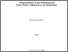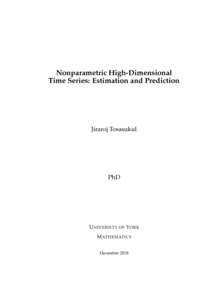# Nonparametric High-Dimensional Time Series: Estimation and Prediction

Tosasukul, Jiraroj (2018) Nonparametric High-Dimensional Time Series: Estimation and Prediction. PhD thesis, University of York.Preview
Text
Thesis of Jiraroj Tosasukul.pdf - Examined Thesis (PDF)

## Abstract

This thesis introduces a new class of functional-coefficient time series models, where the regressors consist of autoregressors and latent factor regressors, and the coefficients are allowed to change with certain index variable. The unobservable factor regressors are estimated through imposing an approximate factor model on very high dimensional exogenous time series variables and subsequently implementing the classical principal component analysis. With the estimated factor regressors, a local linear smoothing method is used to estimate the coefficient functions and obtain a one-step ahead nonlinear forecast of the response variable, and then a wild bootstrap procedure is introduced to construct the prediction interval. The developed methodology is further extended to the case of multivariate response vectors and the model is generalised to the factor-augmented vector time series model with functional coefficients. The latter substantially generalises the linear factor-augmented vector autoregressive model which has been extensively studied in the literature. Under some regularity conditions, the asymptotic properties of the proposed methods are derived. In particular, we show that the local linear estimator and the nonlinear forecast using the estimated factor regressors are asymptotically equivalent to those using the true latent factor regressors. The latter is not feasible in practical applications. This thesis also discusses selection of the numbers of autoregressors and factor regressors and choice of bandwidth in local linear estimation. Some simulation studies and an empirical application to predict the UK inflation are given to investigate the performance of our model and methodology in finite samples.

Item Type: Thesis (PhD) The University of York > Mathematics (York) uk.bl.ethos.778888 Mr Jiraroj Tosasukul 04 Jun 2019 13:49 19 Feb 2020 13:08 http://etheses.whiterose.ac.uk/id/eprint/23863

### Available Versions of this Item

• Nonparametric High-Dimensional Time Series: Estimation and Prediction. (deposited 04 Jun 2019 13:49) [Currently Displayed]